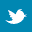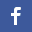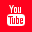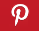Completely Solved C, C++ Programs Assignment.Quick Search Database Videos Tutorials Ebooks Forums FAQ Aboutus Household Industrial Manufacturing Service Shopping Transportation### C program to check whether a number is Armstrong number or not.

 Filed Under: C Assignments

Problem statement:
Write a program to check whether a number is Armstrong number or not.
Theory:
An Armstrong number is an n digit base b such that the sum of its (base b) digit raised to the power n is the number itself.
An example of Armstrong number is 371,i.e 3**3+7**3+1**3=371.

Algorithm:-
/*an algoithm to check a number is armstrong or not*/
procedure armstrng_num(n)
Begin armstrng_num
for t=n to 1 do
rem<-t%10
sum<-sum+cube(rem)
t<-t/10
end for
if sum=n
return(true)
end if

End armstrng_num

PROGRAM LISTING:
#include<stdio.h>
main()
{
int n,rem,sum,temp;
sum=0;
printf("enter a numbern");
scanf("%d",&n);
temp=n;
while(rem!=0)
{
n=n/10;
rem=n%10;
sum=sum+(rem*rem*rem);
}
if(sum==temp)
printf("the number %d is Armstrong",temp);
else
printf("the number %d is not Armstrong",temp);
}

Output:
enter a number 153
the number 153 is Armstrong

Discussion:
• Armstrong number is the summation of its own digits each raised to the power of the number of digits.
• A While loop is used for extracting the digits as well as getting the sum of their cube.
• An Armstrong Number of three digits is an integer such that the sum of the cubes of its digits is equal to the number itself. For example , 371 is an Armstrong number since 3^3+7^3+1^3=371.Back to main directory:Software Practical